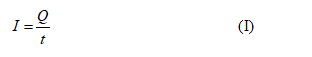# If a current of 1.28 amps moves in a wire, how many electrons pass by any point in the wire each second?  Express your answer in exponential notation.

Question
1 views

If a current of 1.28 amps moves in a wire, how many electrons pass by any point in the wire each second?  Express your answer in exponential notation.

check_circle

Step 1 Concept

Current is defined as the rate of flow of charge.The net charge in a system is depends on the number of charge carriers, and the charge of a single electron is 1.6x10-19C

...

### Want to see the full answer?

See Solution

#### Want to see this answer and more?

Solutions are written by subject experts who are available 24/7. Questions are typically answered within 1 hour.*

See Solution
*Response times may vary by subject and question.
Tagged in

### Physics